Subject:
Numbers and Operations
Material Type:
Lesson Plan
Level:
Middle School
7
Provider:
Pearson
Tags:
Language:
English
Media Formats:
Text/HTML

# Education Standards## Overview

Students use the Hot Air Balloon interactive to model integer addition. They then move to modeling addition on horizontal number lines. They look for patterns in their work and their answers to understand general addition methods.

# Key Concepts

To add two numbers on a number line, start at 0. Move to the first addend. Then, move in the positive direction (up or right) to add a positive integer or in the negative direction (down or left) to add a negative integer.

Here is −6 + 4 on a number line: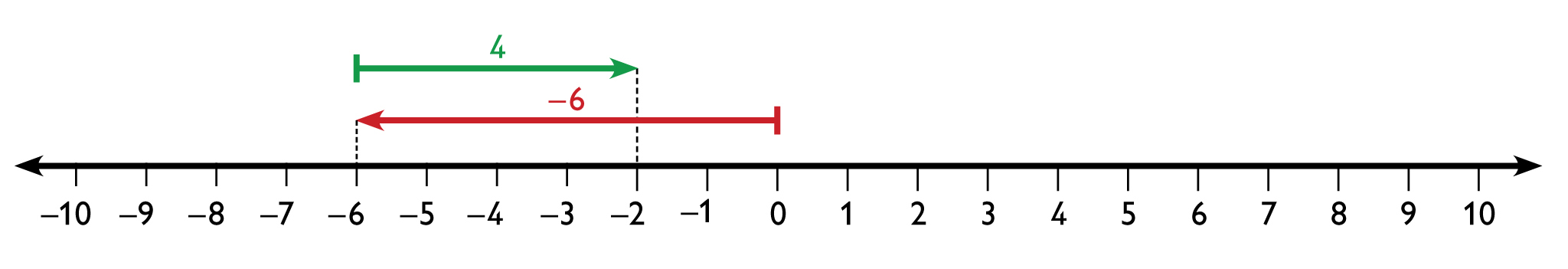The rule for integer addition (which extends to addition of rational numbers) is easiest to state if it is broken into two cases:

• If both addends have the same sign, add their absolute values and give the result the same sign as the addends. For example, to find −5 + (−9), first find |−5|  +|−9| = 14. Because both addends are negative the result is negative. So, −5 + (−9) = −14.
• If the addends have different signs, subtract the lesser absolute value from the greater absolute value. Give the answer the same sign as the addend with the greater absolute value. For example, to find 5 + (−9), find |−9| − |5| = 9 − 5 = 4. Because −9 has the greater absolute value, the result is negative. So, 5 + (−9) = −4.

# Goals and Learning Objectives

• Model integer addition on a number line.
• Learn general methods for adding integers.

# Teacher Demo

Demonstrate how to use the Hot Air Balloon interactive to model −4 + 9 as students follow along:

• Put the balloon at 0.
• To model the first addend, −4, add 4 units of weight and move the balloon.
• To model adding positive 9, add 9 units of heat and move the balloon.

The position of the balloon will be 5. So, −4 + 9 = 5.

# Lesson Guide

Have students solve the remaining addition problems in the Opening. Remind them before each problem to put the balloon back at 0. Briefly review the answers.

• −4 + 9 = 5
• 7 + (−7) = 0
• −3 + (−6) = −9
• 2 + 8 = 10

# Hot Air Balloon

The Hot Air Balloon interactive can be used to model addition of integers. In the simulation, positive integers are represented by heating the air and negative integers are represented by weight.

For example, to model adding −4 + 9, start at 0, add 4 units of weight, and then add 9 units of heat.

Use the Hot Air Balloon interactive to model each problem and find the sum. Write your answer as an equation.

• −4 + 9
• 7 + (−7)
• −3 + (−6)
• 2 + 8

INTERACTIVE: Hot Air Balloon

# Lesson Guide

Briefly discuss modeling integer addition on a vertical or horizontal number line.

# On a Number Line

Discuss the following with your classmates.

You can also model addition of integers on a horizontal or vertical number line.

Just as with the balloon model:

• Adding a positive number (heat) means moving in a positive direction (up or to the right).
• Adding a negative number (weight) means moving in a negative direction (down or to the left).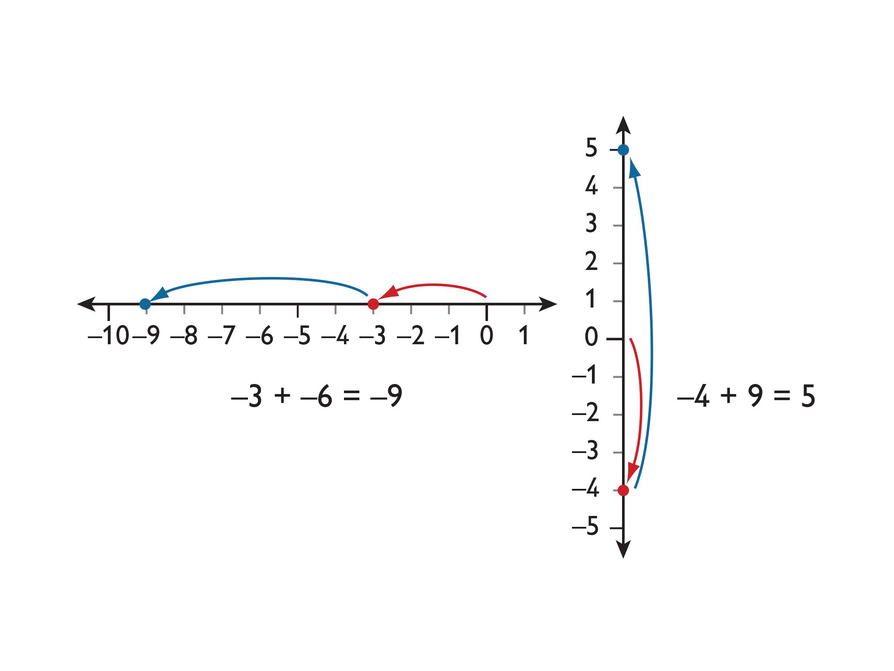# Lesson Guide

Discuss the Math Mission. Students will model integer addition on a number line and analyze guidelines for adding integers.

## Opening

Model integer addition on a number line and analyze guidelines for adding integers.

# Preparing for Ways of Thinking

Have students work independently so they can become familiar with the Number Line Tool.

# Interventions

Student has difficulty modeling addition problems.

• Be sure to start at 0.
• Draw an arrow to show the first addend. In which direction should it point? How long should it be?
• For the second arrow, start where the first arrow ends.
• Look at the second addend. In which direction should the arrow point for that addend? How long should it be?
• Where does the second arrow end? That value is the sum.

• 3 + 3 = 6
•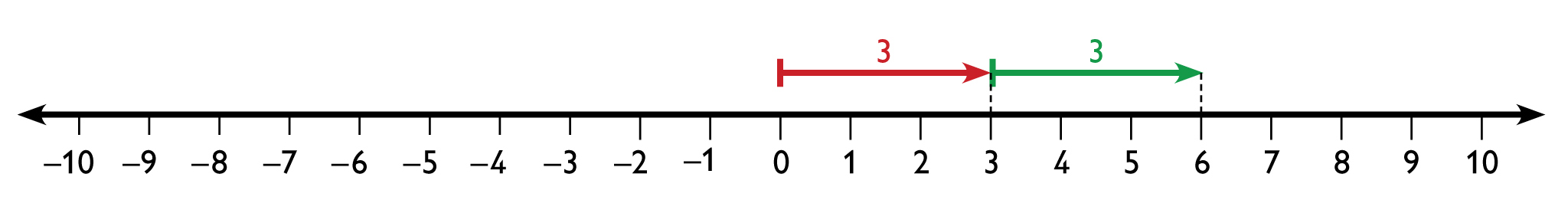• –6 + 4 = –2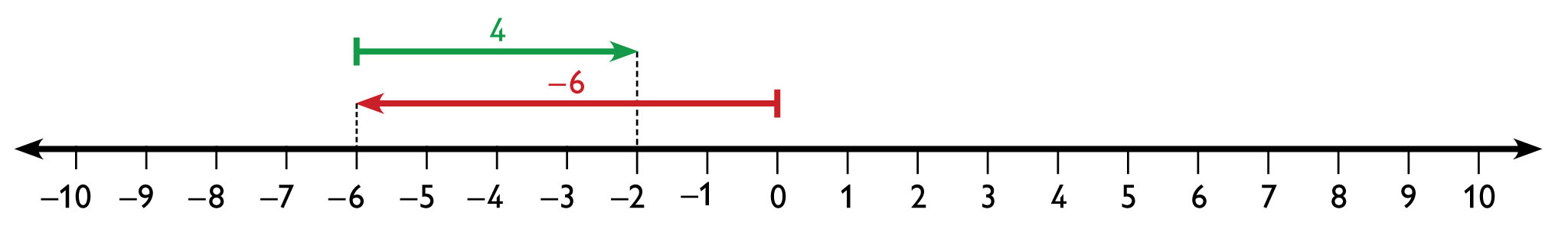• 5 + (–9) = –4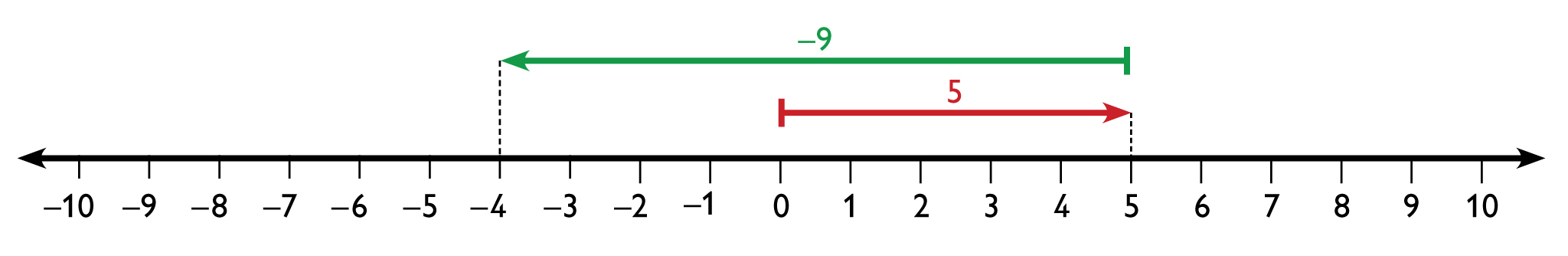• –4 + (–2) = –6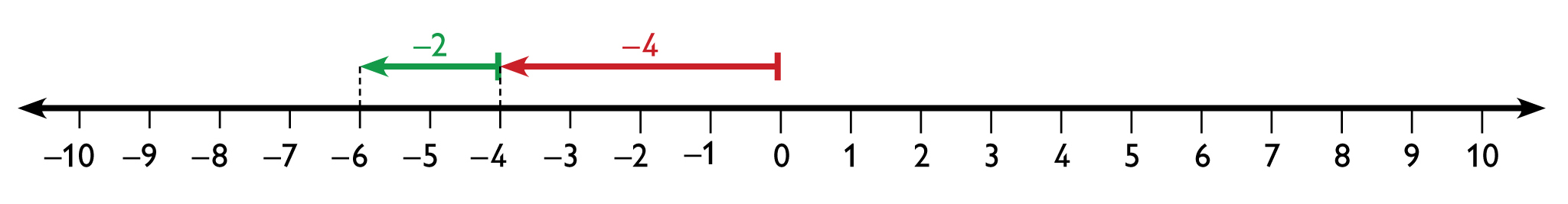• –2 + 7 = 5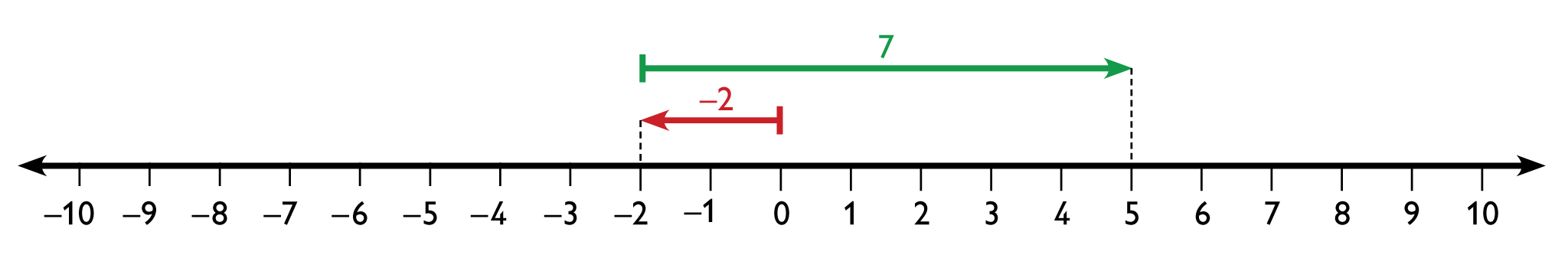• 8 + (–8) = 0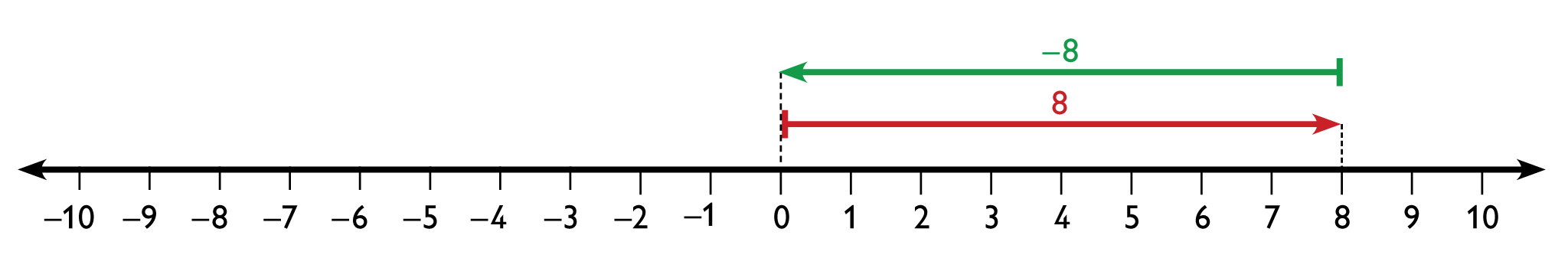# Add Integers on a Number Line

Model each addition problem on using the Number Line Tool.

• 3 + 3
• –6 + 4
• 5 + (–9)
• –4 + (–2)
• –2 + 7
• 8 + (–8)

INTERACTIVE: Number Line Tool

## Hint:

Remember to start the arrow for the first addend at 0. How can you figure out which direction the arrow should face? Where should you start the arrow for the second addend?

# Lesson Guide

Have students work in pairs or small groups when working through the generalizations presented by Jack, Marcus, and Lucy.

ELL: When forming partnerships, consider the task at hand. Since part of the task is language heavy, pair ELLs with native speakers that can help them understand the generalizations.

SWD: Have students who easily understand the task demonstrate their understanding of the generalizations to struggling students. This promotes cooperative learning and is beneficial to both the stronger student and the student who is striving to learn the concept.

# Mathematics

Each of the statements made by Jack, Marcus, and Lucy offer a general method for adding positive and negative integers.

Jack’s statement is an example of Common Core State Standard 7.NS.1.b: “Understand $p+q$ as the number located a distance $|q|$ from $p$, in the positive or negative direction depending on whether $q$ is positive or negative.” It is a method for adding integers using a number line.

Marcus’s statement provides a method for determining the sign of the sum.

Lucy’s statement provides a method for adding integers without using a number line.

# Mathematical Practices

Mathematical Practice 2: Reason abstractly and quantitatively.

Students reason quantitatively about the specific addition problems, and then reason abstractly to explain the statements made by Jack, Marcus, and Lucy. For example, they may reason that adding any two numbers with the same sign joins the arrows for the numbers end-to-end, so the “length” of the sum is the sum of the lengths (absolute values) of the addends and the direction is the same as those of the addends.

Mathematical Practice 8: Look for and express regularity in repeated reasoning.

Students look for patterns in the numerical equations and number line models to draw conclusions about the validity of the general statements made by Jack, Marcus, and Lucy.

The generalizations made by Jack, Marcus, and Lucy are all correct. Explanations will vary.

## Work Time

Jack's generalization:

You can use the number line to show $a+b$, where $a$ and $b$ are any two numbers:

• Start at $a$ (which may be positive, negative, or 0).
• From $a$, move a distance of $|b|$ to the right if $b$ is positive or to the left if $b$ is negative. The number you arrive at on the number line is the answer to $a+b$.

Marcus's generalization:

• The sum $a+b$ is negative if both $a$ and $b$ are negative or if the negative number is a greater distance from 0 (has a greater absolute value) than the positive number.
• The sum $a+b$ is positive if both $a$ and $b$ are positive or if the positive number is a greater distance from 0 (has a greater absolute value) than the negative number.

Lucy's generalization:

• When you add two numbers with the same sign, add their absolute values and write the sum using the sign of the numbers.
• When you add two numbers with different signs, find the difference between their absolute values and write the difference using the sign of the number that has the larger absolute value.

Who is correct? Explain why.

## Hint:

Look at your equations and number lines from the previous problems.

• Apply each student’s generalization to your work. Does the generalization work?
• Think about how you created each number line. How did the signs of the addends determine what you did on the number line?

# Lesson Guide

Look for students who make clear connections between the numerical problems, number lines, and generalizations to share during Ways of Thinking. Students should be able to defend their analyses and support them with examples.

ELL: Provide ELLs the opportunity to represent their thinking using number lines if they wish to be sure that language is not getting in the way of communicating their understanding.

# Challenge Problem

• If both addends are positive or if the positive addend has a greater absolute value than the negative addend, the sum will be positive.
• If both addends are negative or if the negative addend has a greater absolute value than the positive addend, the sum will be negative.

# Prepare a Presentation

Analyze the generalizations made by Jack, Marcus, and Lucy. For each one, state whether you agree or disagree. Explain your thinking. Support your explanations with the equations and number lines you worked on earlier in the lesson.

# Challenge Problem

• How can you tell that the sum of two integers will be positive by just looking at the addends?
• How can you tell that the sum will be negative by just looking at the addends?

# Lesson Guide

Have students present their explanations.

Point out that Lucy’s statement gives us rules for adding positive and negative integers. Have the class rewrite the rules in their own words, drawing from the student presentations.

Write the following problems on the board and ask students to find the sums:

• 9 + (–9)
• –17 + 17
• 624 + (–624)
• –49 + 49

Students will find that all the sums are 0. Ask if anyone can summarize the pattern in these problems and answers. If necessary, help students state that the sum of any integer and its opposite is 0. Tell students that we sometimes refer to the opposite of a number as its additive inverse.

# Mathematical Practices

Mathematical Practice 6: Attend to precision.

Students should explain their analyses and give examples to support them. As each presenter explains, other students should critique, ask questions, and help to clarify ideas. Work as a class to correct incorrect concepts.

# Ways of Thinking: Make Connections

## Hint:

• How did you know which direction to go for the second addend?
• Can you explain how you determined the sign of your answer?
• How would you compare adding a negative number with subtracting?

# Lesson Guide

Have students work individually on the problems. They should choose one or more of the methods proposed by Jack, Marcus, and Lucy to solve the problems.

SWD: Some students may struggle with the task of writing a summary of the mathematics from the lesson. Possible supports:

• Prior to writing the summary, have students discuss their ideas with a partner or adult and rehearse what they might write.
• Allow students to map out their ideas in outline form or in a concept web.

• –12 + 7 = –5
• 29 + (–18) = 11
• –74 + (–38)  = –112
• 324 + (–324) = 0

## Work Time

Solve the problems. Write each solution as an equation.

• –12 + 7
• 29 + (–18)
• –74 + (–38)
• 324 + (–324)

# A Possible Summary

To add integers $a$ and $b$ using a number line, start at $a$. If $b$ is positive, then move a distance $|b|$ to the right. If $b$ is negative, move a distance $|b|$ to the left.

You can also add integers without a number line. If both numbers are positive or both numbers are negative, ignore the sign and add them together. The sum will be the same sign as the addends. If the addends have a different sign, subtract the smaller from the larger. The sum will be the same sign as the addend with the greater absolute value.

# Summary of the Math: Add Integers

## Hint:

• Do you describe how to add integers on a number line?
• Do you explain how to determine the sign of the answer?